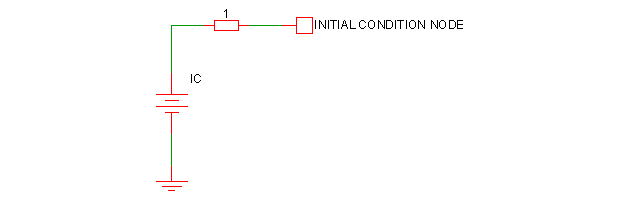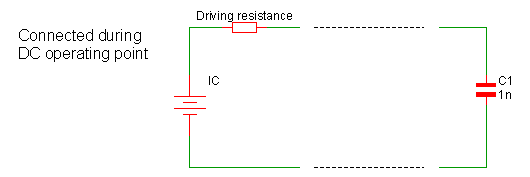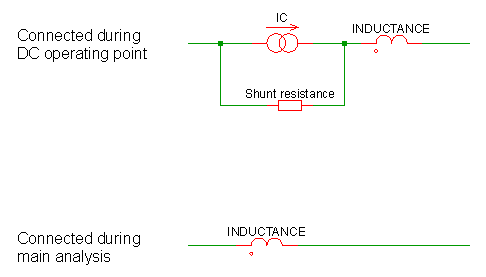# Initial Conditions

Initial conditions may be applied to capacitors and inductors and may also be applied to a single node using the .IC statement. Initial conditions force a voltage or current to be applied during the DC operating point analysis. Here we describe the various methods to apply an initial condition.

In this topic:

## Node Initial Condition

A voltage initial condition may be applied to a node using the .IC statement, see .IC. This method applies the initial condition through a fixed resistor. In effect the following circuit is applied to the node during the DC operating point then removed when the main analysis starts:The above shows a driving resistance of 1$\Omega$. This is the default value but can be changed using the ICRES option:
.OPTIONS ICRES=1m

The above sets the resistance to 1m$\Omega$.

Note that an initial condition may also be applied to a node or across a pair of nodes using a capacitor with a value of zero. See next section.

## Capacitor Initial Condition

An initial condition may be defined for a capacitor using the IC parameter. The following diagram shows how this is configured:The actual interpretation of the IC parameter depends on the INITCONDMODE option setting:
.OPTIONS INITCONDMODE=0|1|2
It also depends on the BRANCH parameter on the capacitor:
Cxxxx n1 n2 capacitance IC=init_condition BRANCH=0|1

The different configurations have come about because of a need for compatibility with other simulators, namely SIMPLIS, Berkeley SPICE and PSpice.

The following table describes the various configurations:
 IC BRANCH INITCONDMODE Configuration NO 0 X No initial condition applied NO 1 X Initial condition=0V, driving resistance=0$\Omega$ YES 0 0 Berkeley SPICE compatible. Initial condition=IC only if UIC specified on .TRAN statement YES 0 1 SIMPLIS compatible. Initial condition=IC, driving resistance=0$\Omega$ YES 0 2 PSpice compatible. Initial condition=IC, driving resistance=ICRES (default=1$\Omega$) YES 1 X SIMPLIS compatible. Initial condition=IC, driving resistance=0$\Omega$

Note that for full PSpice compatibility, the ICRES option should be set to 1m. Alternatively the option setting PSPICECOMPATIBILITY=1 or PSPICECOMPATIBILITY=2 may be set which sets ICRES to 1m and INITCONDMODE to 2.

## Inductor Initial Condition

An initial condition may be defined for a inductor using the IC parameter. The following diagram shows how this is configured:The actual interpretation of the IC parameter depends on the INITCONDMODE option setting:
.OPTIONS INITCONDMODE=0|1|2
It also depends on the BRANCH parameter on the inductor:
Lxxxx n1 n2 inductance IC=init_condition BRANCH=0|1

The different configurations have come about because of a need for compatibility with other simulators, namely SIMPLIS, Berkeley SPICE and PSpice.

The following table describes the various configurations:
 IC BRANCH INITCONDMODE Configuration NO 1 X No initial condition applied NO 0 X Initial condition=0A, shunt resistance=$\infty$ YES 1 0 Berkeley SPICE compatible. Initial condition=IC only if UIC specified on .TRAN statement YES 1 1 SIMPLIS compatible. Initial condition=IC, shunt resistance=$\infty$ YES 1 2 PSpice compatible. Initial condition=IC, shunt resistance=1e9 $\Omega$ YES 0 X SIMPLIS compatible. Initial condition=IC, shunt resistance=$\infty$